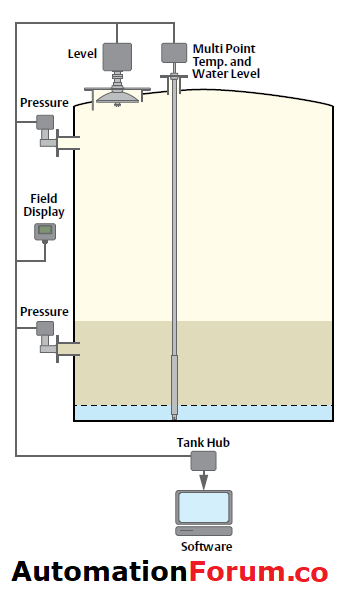# What is Hybrid level measurement system?## What is Hybrid tank gauging system?

Hybrid level measurement system is the combination of traditional tank gauging system and a Hydrostatic Tank Gauging.

Hybrid gauging system is mostly used in petroleum industries, where online measurement of level, density and temperature are required.

## Design of Hybrid gauging system:

To calculate the density of liquid in the tank, pressure sensors are installed in the tank. Therefore, if the tank has atmospheric pressure, the hybrid system has one pressure sensor and two pressure sensors if the tank is not ventilated freely.

Pressure measurement, combined with level, provides true average density measurement over the entire product level height

A typical HTMS system comprises of either a radar or servo gauge and one hydrostatic head measuring pressure transmitter-the fluid product column pressure in tanks. For those tanks where the gas or vapor pressure the pressure in the empty part of the tank is not ambient, for example, in cases where the tank is not open to the atmosphere, an additional pressure transmitter must be added.

### Hydrostatic Tank Gauging:

There is an extra pressure sensor and no level gauge system in the Hydrostatic Tank Gauge (HTG) scheme. Only the density between the P1 and P2 sensors calculates the product density in the HTG scheme.

• It provides direct mass measurement with only pressure transmitters to measure hydrostatic pressure in determining density via a fixed distance and vapour pressure.
• Low-cost solution for mass measurement.

## Level measurement calculation procedure for Hybrid tank measuring system:

• It also starts with the level from the automatic tank gauge (ATG).
• But then, the pressure P1 is also measured (P3 if the tank is non atmospheric)
• The real observed density is continually calculated from the point and pressure.
• The temperature is measured again-but now it is only necessary for the calculation of the VCF and the reference density.
• The largest difference is with the mass, since the mass is now calculated from the GOV and the observed density.
• The Gross Standard Volume (GSV) from the GOV and the VCF is calculated again
• The standard density can also be calculated through the tables of the API / ASTM, but is now a continually measured value rather than a grab sample.

## Advantages of Hybrid level measurement system:

• Continuous density measurement
• Mass assessment independent of the temperature assessment, meaning that the measurement quality is not dependent on the way the product temperature is measured.
• Possibility of continuous standard density calculation
• No performance loss for conventional volume assessment

Scroll to Top